# Algebra 1 - Inequalities & Graphing

## Introduction

• An inequality is a mathematical statement in which two real numbers or algebraic expressions are compared using four symbols "$<$", "$>$", "$\le$", "$\ge$".

## Classification of Inequalities

• Inequalities can be classified based on factors such as the type of inequality sign, number of variables, degree of variables in the inequality, etc. Some of the important types of inequalities worth remembering are described below:

Strict Inequality & Slack Inequality

• An inequality involving only "less than $\left(<\right)$" or "greater than $\left(>\right)$" symbols is called Strict Inequality. Example: $9>2$ (9 is strictly greater than 2), $2<10$ (2 is strictly less than 10), etc.
• An inequality involving "less than equal to $\left(\le \right)$" or "greater than equal to $\left(\ge \right)$" symbols is Slack Inequality. Example: $x\ge 2$ ($x$ is greater than or equal to 2), $x\le 12$ ($x$ is less than or equal to 12), etc.

Linear inequality & Non-linear Inequality

• An inequality in which the degree (highest power) of the variable is one is called Linear Inequality. Example: $5x-2>15$ (degree of the variable $x$ is 1),  $2x+3y\ge 5$ (degree of both the variables $x$ & $y$ is 1), etc.
• An inequality in which the degree (highest power) of the variable is anything other than one is called Non-linear Inequality. Example: ${x}^{2}-4x+4\le 16$ (degree of the variable is 2), ${x}^{3}-2x+7\ge 33$ (degree of the variable is 3), etc.

Linear inequality in one variable & two variables:

• An inequality in which the degree (highest power) of the variable is one & contains terms only in a single variable is called Linear Inequality in one variable. Example: $2x-2\ge 5x-15$ (single variable $x$ & degree of the variable is 1), $8y-2>22$ (single variable $y$ & degree of the variable is 1), etc.
• An inequality in which the degree (highest power) of the variable is one & consists of two variables is called Linear Inequality in two variables. Example: $2x-4\le 4y+6$ (two variables  & degree of both the variables is 1), $15z-2t<120$ (two variables  & degree of both the variables is 1), etc.

## Properties of Inequalities

• The properties of Inequalities are listed below:

• It states that "adding the same number to each side of an inequality produces an equivalent inequality wherein the sign of inequality remains unaffected".

Subtraction Property:

• It states that "subtracting the same number from both sides of an inequality results in an equivalent inequality wherein the sign of inequality remains unaffected".

Multiplication Property:

• It states that "multiplying both sides of an inequality by a positive number results in an equivalent inequality wherein the sign of inequality remains unaffected".
• It also states that "multiplying both sides of an inequality by a negative number results in a new inequality wherein the sign of inequality is reversed".

Division Property:

• It states that "dividing both sides of an inequality by a positive number results in an equivalent inequality wherein the sign of inequality remains unaffected".
• It also states that "dividing both sides of an inequality by a negative number results in a new inequality wherein the sign of inequality is reversed".

## Solving & Graphing linear inequalities

• The solution of linear inequalities in one variable and linear inequalities in two variables is described below.

## Algebraic Solutions of Linear Inequalities in One Variable and their Graphical Representation

Algebraic Solutions of Linear Inequalities in One Variable

• We can solve linear inequalities in one variable using the properties of linear inequalities already mentioned. We can summarize the steps involved in solving linear inequalities in the form of rules as mentioned below:
• Rule 1: The same number may be added to or subtracted from both sides of an inequality without affecting the sign of the inequality.
• Rule 2: Both sides of an inequality can be multiplied or divided by the same positive number without affecting the sign of the inequality. But when both sides are multiplied or divided by a negative number, then the sign of the inequality gets reversed.

Graphical Representation Linear Inequalities in One Variable

• Linear inequalities in one variable can be visualized on a number line. Plotting linear inequalities in one variable involves the following steps:
• Solve the linear inequality to find the variable's value that satisfies the inequality.
• Locate and mark the solution on the number line.
• We use an open dot for strict inequalities to indicate that the point is not part of the solution.
• We use a closed dot for slack inequalities to indicate that the point is part of the solution.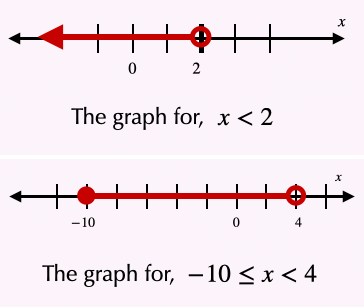## Graphical Solution of Linear Inequalities in Two Variables

• The graphical representation of linear inequalities in two variables is closely related to the graphs of linear equations.
• We know that a linear equation in two variables has infinitely many solutions & forms a straight line when graphed. On the other hand, a linear inequality in two variables has a solution set consisting of a region either above or below this equality line.
• The boundary may or may not be included in the solution set.
• In the case of a strict inequality (involving $<$ and $>$), we use a dashed line to indicate that the boundary is not included.
• In the case of a slack inequality (involving $\le$ and $\ge$), we use a solid line to indicate that the boundary is included.

The graphs of linear inequalities in two variables of the four cases are mentioned below:

1) $\mathbit{y}\mathbf{>}\mathbit{a}\mathbit{x}\mathbf{+}\mathbit{b}$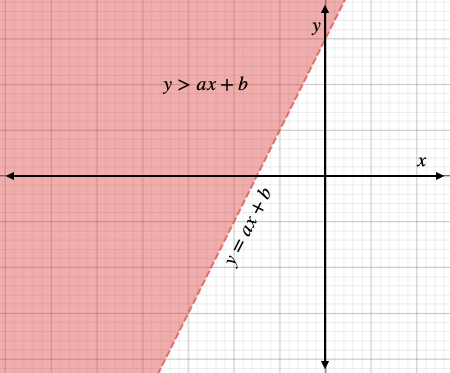• The dashed line is not a part of the solution set.

2) $\mathbit{y}\mathbf{<}\mathbit{a}\mathbit{x}\mathbf{+}\mathbit{b}$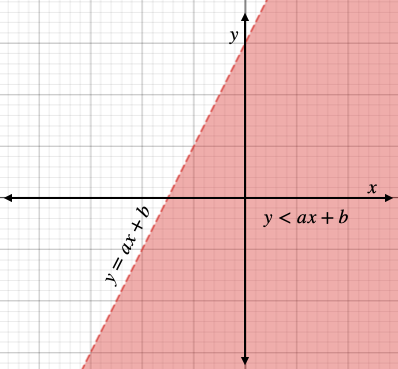• The dashed line is not a part of the solution set.

3) $\mathbit{y}\mathbf{\ge }\mathbit{a}\mathbit{x}\mathbf{+}\mathbit{b}$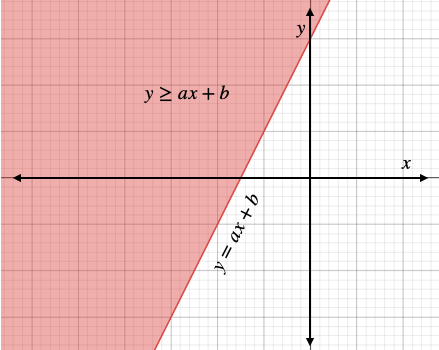• The solid line is a part of the solution set.

4) $\mathbit{y}\mathbf{\le }\mathbit{a}\mathbit{x}\mathbf{+}\mathbit{b}$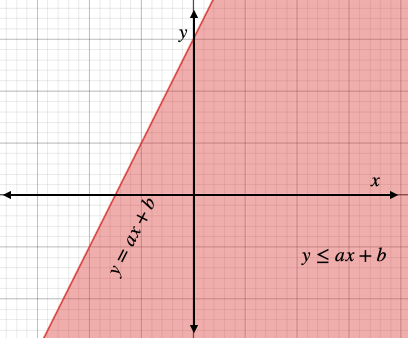• The solid line is a part of the solution set.

## Solved Examples

Example 1: Solve the inequality.

$\mathbf{2}\mathbit{x}\mathbf{-}\mathbf{7}\mathbf{>}\mathbf{5}$

Solution: $2x-7>5$

$2x-7+7>5+7$

$2x>12$

$\frac{2}{2}x>\frac{12}{2}$

$x>6$

Example 2: Solve the inequality.

$\mathbf{8}\mathbit{x}\mathbf{+}\mathbf{13}\mathbf{<}\mathbf{29}$

Solution: $8x+13<29$

$8x+13-13<29-13$

$8x<16$

$\frac{8}{8}x<\frac{16}{8}$

$x<2$

Example 3: Solve the inequality.

$\mathbf{10}\mathbit{x}\mathbf{-}\mathbf{55}\mathbf{\ge }\mathbf{15}$

Solution: $10x-55\ge 15$

$10x-55+55\ge 15+55$

$10x\ge 70$

$\frac{10}{10}x\ge \frac{70}{10}$

$x\ge 7$

Example 4: Solve the inequality.

$\mathbf{3}\mathbit{x}\mathbf{-}\mathbf{12}\mathbf{\le }\mathbf{12}$

Solution: $3x-12\le 12$

$3x-12+12\le 12+12$

$3x\le 24$

$\frac{3}{3}x\le \frac{24}{3}$

$x\le 8$

Example 5: Solve the inequality.

$\mathbf{-}\mathbf{9}\mathbf{<}\mathbf{2}\mathbit{x}\mathbf{+}\mathbf{1}\mathbf{<}\mathbf{15}$

Solution: $-9<2x+1<15$

$-9-1<2x+1-1<15-1$

$-10<2x<14$

$\frac{-10}{2}<\frac{2}{2}x<\frac{14}{2}$

$-5

Example 6: Solve the inequality.

$\mathbf{-}\mathbf{1}\mathbf{<}\frac{\mathbf{5}\mathbf{x}\mathbf{-}\mathbf{7}}{\mathbf{2}}\mathbf{\le }\mathbf{5}$

Solution: $-1<\frac{5\mathrm{x}-7}{2}\le 5$

$-1×2<\frac{5\mathrm{x}-7}{2}×2\le 5×2$

$-2<5\mathrm{x}-7\le 10$

$-2+7<5\mathrm{x}-7+7\le 10+7$

$5<5\mathrm{x}\le 17$

$\frac{5}{5}<\frac{5}{5}\mathrm{x}\le \frac{17}{5}$

$1<\mathrm{x}\le \frac{17}{5}$

Example 7: Solve the inequality.

$\mathbf{4}\mathbf{×}\mathbf{\left(}\mathbf{-}\mathbf{2}\mathbf{x}\mathbf{+}\mathbf{9}\mathbf{\right)}\mathbf{>}\mathbit{x}\mathbf{+}\mathbf{1}$

Solution: $4×\left(-2\mathrm{x}+9\right)>x+1$

$-8\mathrm{x}+36>x+1$

$-8\mathrm{x}+36-36>x+1-36$

$-8\mathrm{x}>x-35$

$-8\mathrm{x}-\mathrm{x}>x-35-x$

$-9\mathrm{x}>-35$

$9\mathrm{x}<35$

$\frac{9}{9}\mathrm{x}<\frac{35}{9}$

$\mathrm{x}<\frac{35}{9}$

Example 8: Plot the inequality.

$\frac{\mathbf{2}\mathbf{x}\mathbf{-}\mathbf{9}}{\mathbf{3}}\mathbf{>}\mathbf{7}$

Solution: First, we need to solve the given inequality

$\frac{2\mathrm{x}-9}{3}>7$

$\frac{2\mathrm{x}-9}{3}×3>7×3$

$2\mathrm{x}-9>21$

$2\mathrm{x}-9+9>21+9$

$2\mathrm{x}>30$

$\frac{2}{2}\mathrm{x}>\frac{30}{2}$

$x>15$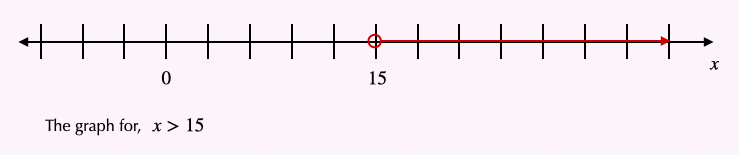Example 9: Plot the inequality.

$\mathbf{-}\mathbf{6}\mathbf{\le }\mathbit{x}\mathbf{-}\mathbf{1}\mathbf{\le }\mathbf{24}$

Solution: First, we need to solve the given inequality

$-6\le x-1\le 24$

$-6+1\le x-1+1\le 24+1$

$-5\le x\le 25$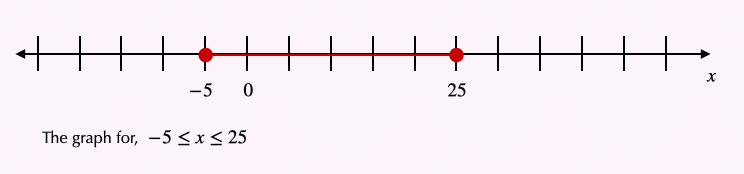Example 10: Graph the solution set of the given inequality in two variables.

$\mathbf{3}\mathbit{x}\mathbf{-}\mathbf{2}\mathbit{y}\mathbf{\ge }\mathbf{-}\mathbf{5}$

Solution: Here, the boundary is represented by the equation $3x-2y=-5$. Since the inequality is not a strict type, we graph the boundary using a solid line. Now let us rewrite the given inequality as:

$3x-2y\ge -5$

$3x-2y-3x\ge -5-3x$

$-2y\ge -5-3x$

$y\le \frac{3}{2}x+\frac{5}{2}$

Now we can graph it.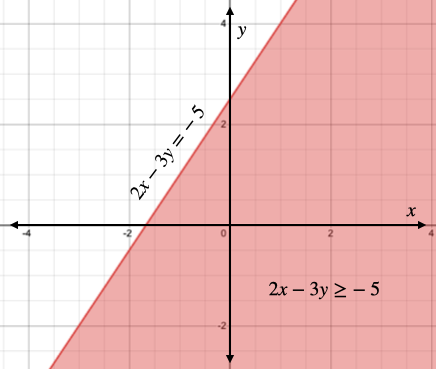The shaded region, including the boundary, represents the solution.

## Cheat Sheet

• Adding the same quantity to both sides of an inequality doesn't change the direction of the inequality sign.
• Likewise, subtracting the same quantity from both sides of an inequality doesn't change the direction of the inequality sign.
• Multiplying both sides of an inequality by a positive number leaves the inequality sign unchanged. In contrast, multiplication by a negative number reverses the direction of inequality.
• Similarly, dividing both sides of an inequality by a positive number leaves the inequality sign unchanged. At the same time, division by a negative number reverses the direction of the inequality.
• Linear inequality in one variable is plotted on a number line. An open circle is used to signify "less than (<)" or "greater than (>)", while a closed circle denotes "less than equal to ($\le$)" or "more than equal to ($\ge$)" type inequality on the number line.
• The solution of linear inequalities in two variables is always a region on the x-y plane in which the boundary may or may not always be included in the solution set.
• We use a dashed line for a strict inequality to indicate that the boundary is not included. On the other hand, for a slacked inequality, we use a solid line to show that it is included

## Blunder Areas

• When solving inequalities, one must not forget to reverse the inequality sign when multiplying or dividing by negative numbers.